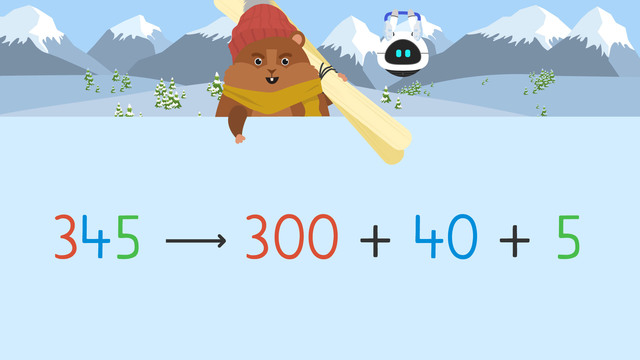# Three Digit Addition with Regrouping - Expanded Form

Content Three Digit Addition with Regrouping - Expanded FormRating

Ø 4.8 / 4 ratings
The authorsTeam Digital
Three Digit Addition with Regrouping - Expanded Form
CCSS.MATH.CONTENT.3.NBT.A.2

## Addition with Expanded Form with Regrouping

What is addition with expanded form with regrouping? This text teaches you how to use expanded form when solving three-digit addition problems (regrouping).Expanded Form is an addition strategy we can use to help solve equations. Expanded form is a way of writing numbers to emphasize the value each digit has. For example, three hundred forty-five can be rewritten in expanded form as three hundred plus forty plus five. We can use this format to help us add with regrouping.

## Addition with Regrouping using Expanded Form – Example

Let’s use the example three hundred eighty one plus ninety-nine.First, rewrite the equation in expanded form, one on top of the other.Then, start adding the ones column. Here the sum is ten. Since ten has zero ones and one ten, we write zero in the ones place. Then, we regroup, or move, the ten to the top of the tens place.Next, add the tens column. Here the sum is one hundred eighty. One hundred eighty has one hundred and eighty tens, so we write eighty in the tens place. Then, we regroup one hundred to the top of the hundreds place.Now, add up the hundreds column and write the sum, four hundred, below.Finally, take the answer out of expanded form by adding it together.## Addition with Regrouping using Expanded Form – Summary of Steps

These are the necessary steps for addition with regrouping using the expanded form.

Step # What to do
1 Rewrite the equation in expanded form, one on top of the other.
2 Add the ones column. If the sum has a ten, regroup and
write it above the tens place.
Then, write the ones value in the ones place.
3 Add the tens column. If the sum has a hundred,
write that above the hundreds place.
Then, write the value of the ten in the tens place.
4 Add up the hundreds column and
write the sum below in the hundreds place.
5 Take the answer out of expanded form by adding it together.

Have you practiced yet? On this website, you can also find three-digit expanded form addition with regrouping examples on worksheets and exercises.

## Three Digit Addition with Regrouping - Expanded Form exercise

Would you like to apply the knowledge you’ve learned? You can review and practice it with the tasks for the video Three Digit Addition with Regrouping - Expanded Form.
• ### Use place value to find the value of the 5.

Hints

What place is the 5 in?

Remember to think about the expanded form place value: Hundreds, Tens, and Ones. These place value blocks represent 257.

Solution

The value of the 5 is 50, since it is in the tens place. When we expand this number, we get 200 + 50 + 7.

• ### What's the correct equation in expanded form?

Hints

Remember, expanded form is showing the numbers in hundreds, tens, and ones. Line up both numbers based on place value.

Here's an example of 430 in expanded form.

Solution

134 + 78 are added like this in expanded form. The hundreds, tens, and ones place line up.

• ### Help solve this problem in expanded form.

Hints

Remember, create the expanded form first, then add starting at the ones column. Regroup as needed.

Look at this example to remember how to regroup based on place value.

Solution

• First, put the numbers in expanded form.
• Then, starting at the ones place, add 3 + 8 to get 11. Since 11 cannot be in the ones column, regroup the 10 into the tens column, and keep the one 1.
• Next, you add the tens column, 10 + 20 + 40 to get 70. No regrouping needs to happen in this step.
• Then, you add the hundreds column, 400 + 200 to get 600.
• Lastly, you add up 600 + 70 + 1 to get 671.

• ### Solve using expanded form.

Hints

Remember, write out the equation in expanded form.

Then, starting from the ones place, add them together. Regroup as needed.

Solution

Together, Imani and Mr. Squeaks tubed for 603 feet! To add 430 + 173:

• First, put the numbers in expanded form.
• Then, starting at the ones place, add 0 + 3 to get 3. No regrouping needs to happen.
• Next, you add the tens column, 30 + 70 to get 100. Since 100 cannot be in the tens column, regroup the 100 into the hundreds column, with 0 tens left.
• Then, you add the hundreds column, 100 + 400 + 100 to get 600.
• Lastly, you add up 600 + 00 + 3 to get 603.

• ### What's the expanded form?

Hints

Remember, expanded form shows hundreds + tens + ones.

Here's an example of 435 in expanded form.

Solution

123 in expanded form is: 100 + 20 + 3.

• ### Find the equation solved correctly in expanded form.

Hints

Remember, add the numbers together using expanded form. Start at the ones place, and regroup as needed.

Check your regrouping in the tens and hundreds columns.

Solution